﻿ 组织信息同步接口-应用集成文档

组织信息同步接口

• 全同步：一次同步全部数据。
• 节点同步：一次同步某个机构节点或者角色节点里的信息。

组织信息同步接口IDataExtraction

• orgId – 机构ID

extractRole

• roleId – 角色ID

extractUserByRole

• roleId – 角色ID

• orgId – 机构ID

其他相关类ExtractResult

ExtractResult是组织信息同步接口中方法的返回值类。

ExtractResult

• data - Map  存储抽取出来的用户数据。

1）增量同步data结构：

Map[Integer, java.util.List[T]]

[key:0 value:增加的数据] [key:1 value:修改的数据] [key:2 value: 删除的数据]

2）全表同步data结构：

Map[Integer, java.util.List[T]]

dataType int 同步数据方式，0:增量 1:全量

组织信息同步相关表结构

机构表(T_org)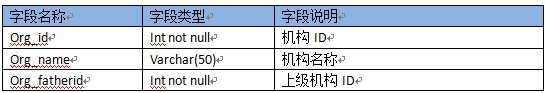角色表(T_role)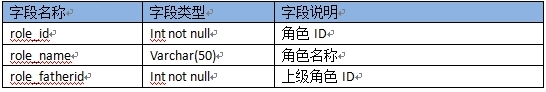用户表（T_user）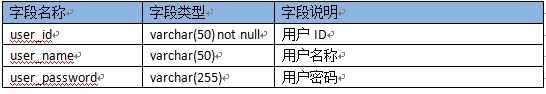机构用户表(RT_orgUser)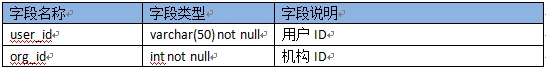角色用户表(RT_roleUser)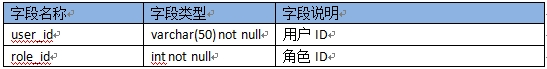组织信息同步接口使用示例

Step2

• customer=com.runqianapp.synchronize.extract.MyDemo1（用于配置IDataExtraction接口实现类）
• listener= com.runqianapp.synchronize.listener.impl.SetPathListener（使用默认即可）
• isSyn=false(是否开启节点树同步功能)

Step3

• <conf_rootorg dataType="1"  default="客户根机构号"  inputType="text"  value="客户根机构号" />
• <conf_rootrole dataType="客户跟角色号" default="1" inputType="text" value="客户跟角色号" />

示例代码

public class MyDemo implements IDataExtraction {

private Connection conn;

public MyDemo() {

try {

//设置数据库连接

Class.forName("oracle.jdbc.driver.OracleDriver");

String url = "jdbc:oracle:thin:@192.168.10.43:1521:ORCL";

String user = "zyzx";

} catch (Exception e) {

e.printStackTrace();

}

}

//全同步时机构抽取方法

public ExtractResult extractOrg() {

String sql = "select c.org_index as org_id,a.org_name,b.org_index as org_father_id from zyzx.sysorg a left join zyzx.sysorgindex b on a.org_parent_id = b.org_id left join zyzx.sysorgindex c on a.org_id = c.org_id";

Map<Integer, Org> orgMap = extractOrg(sql);

return new ExtractResult(orgMap, 1);

}

//节点同步时根据机构id抽取下级机构数据方法

public ExtractResult extractOrg(int orgId) {

String sql = "select c.org_index as org_id,a.org_name,b.org_index as org_father_id from zyzx.sysorg a left join zyzx.sysorgindex b on a.org_parent_id = b.org_id left join zyzx.sysorgindex c on a.org_id = c.org_id where b.org_index="+orgId;

Map<Integer, Org> orgMap = extractOrg(sql);

if(orgMap.size()<1){

return new ExtractResult();

}

return new ExtractResult(orgMap, 1);

}

//处理机构字段，转换成所要求的机构类对象

private Map<Integer, Org> extractOrg(String sql) {

Map<Integer, Org> orgMap = new HashMap<Integer, Org>();

Statement stmt = null;

ResultSet rs = null;

try {

stmt = conn.createStatement();

rs = stmt.executeQuery(sql);

while(rs.next()) {

orgMap.put(rs.getInt(1), new Org(rs.getInt(1), rs.getString(2), rs.getInt(3)));

}

} catch (SQLException e) {

e.printStackTrace();

} finally {

close(rs, stmt);

}

return orgMap;

}

//抽取根机构数据

public Org extractRootOrg() {

Org org = null;

Statement stmt = null;

ResultSet rs = null;

String sql = "select c.org_index as org_id,a.org_name,b.org_index as org_father_id from zyzx.sysorg a left join zyzx.sysorgindex b on a.org_parent_id = b.org_id left join zyzx.sysorgindex c on a.org_id = c.org_id where a.org_id='8a9e00d62c30bbf5012c61f2039e0648'";

try {

stmt = conn.createStatement();

rs = stmt.executeQuery(sql);

if(rs.next()) {

org = new Org(rs.getInt(1), rs.getString(2), rs.getInt(3));

}

} catch (SQLException e) {

e.printStackTrace();

} finally {

close(rs, stmt);

}

return org;

}

//其他用户，角色抽取，机构用户关系，角色用户关系部分代码类似，略

……